Ex 5.6

Chapter 5 Class 12 Continuity and Differentiability
Serial order wise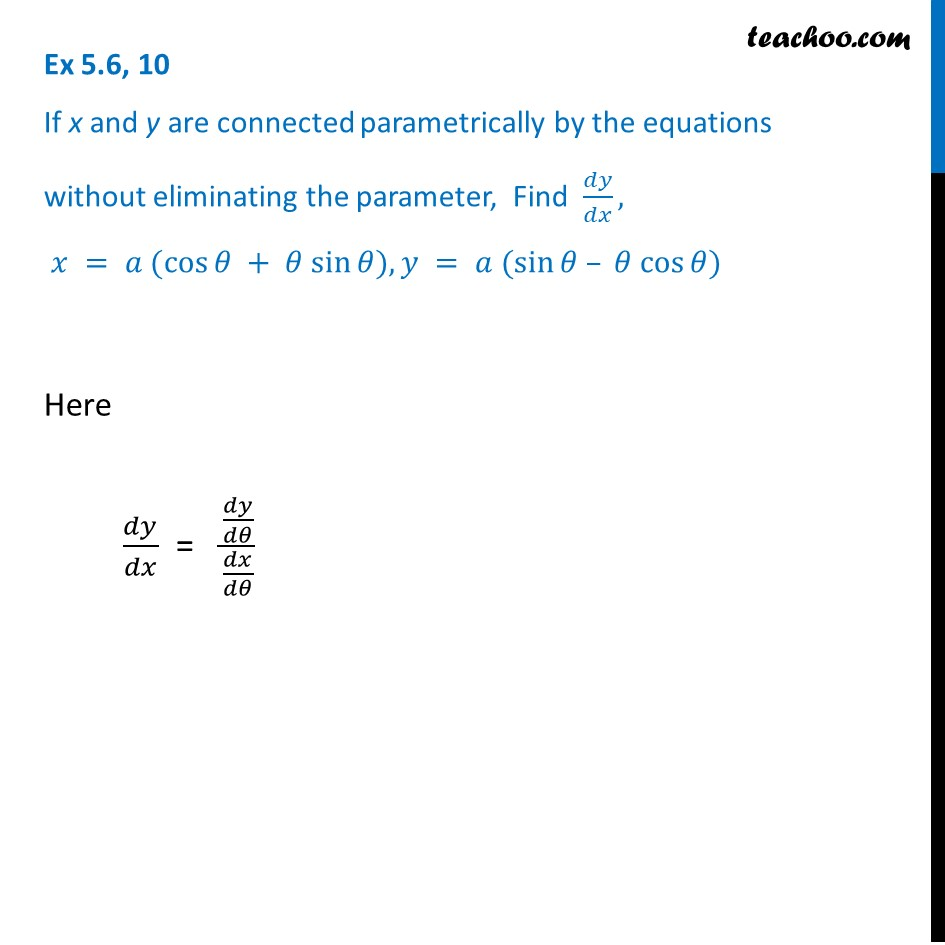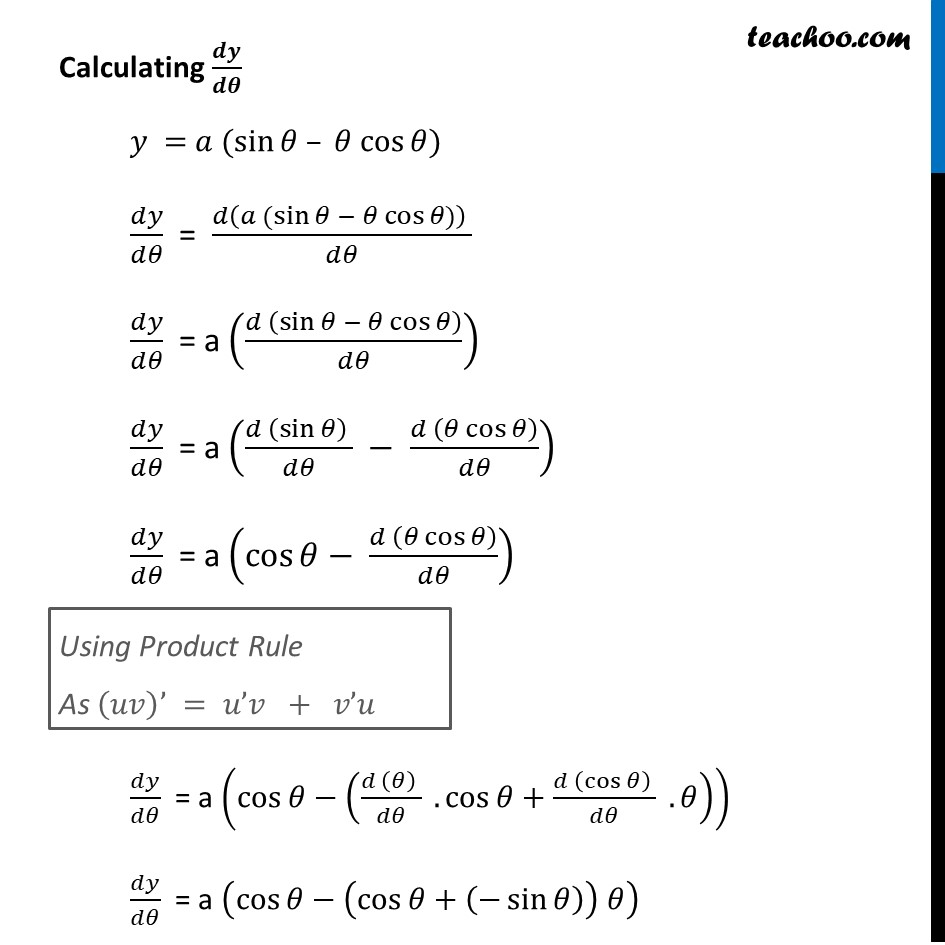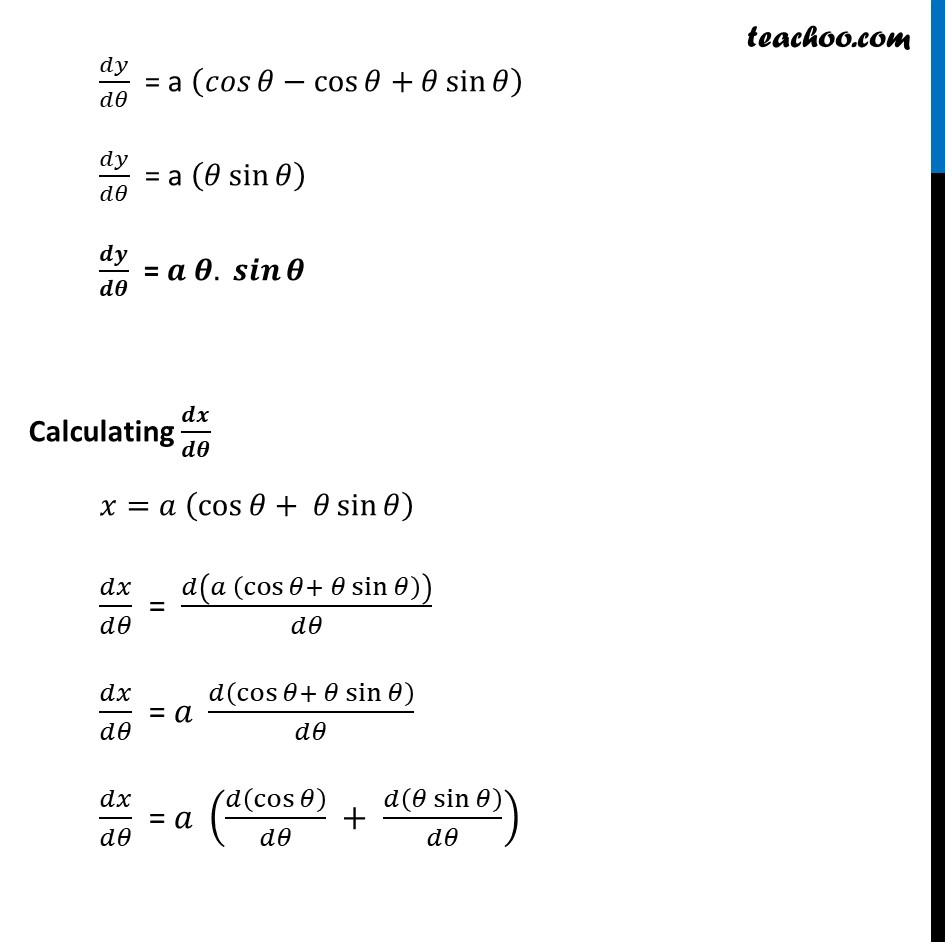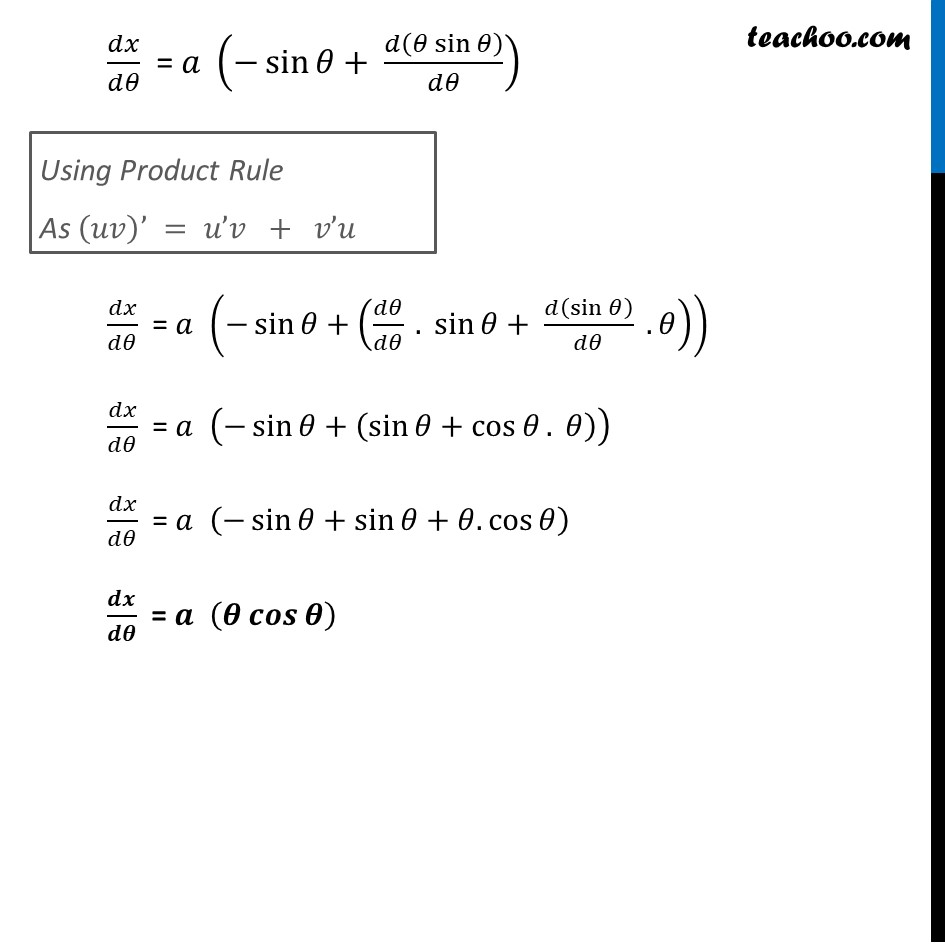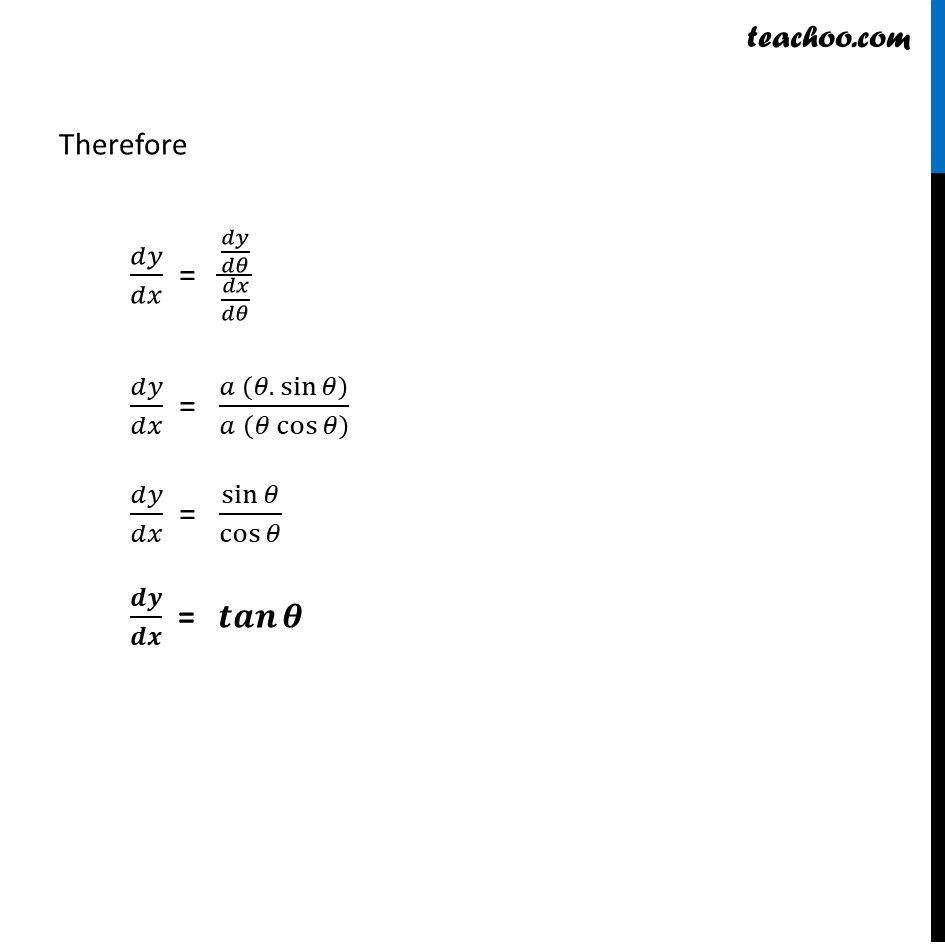Learn in your speed, with individual attention - Teachoo Maths 1-on-1 Class

### Transcript

Ex 5.6, 10 If x and y are connected parametrically by the equations without eliminating the parameter, Find 𝑑𝑦/𝑑𝑥, 𝑥 = 𝑎 (cos⁡𝜃 + 𝜃 sin⁡𝜃), 𝑦 = 𝑎 (sin⁡𝜃 – 𝜃 cos⁡𝜃)Here 𝑑𝑦/𝑑𝑥 = (𝑑𝑦/𝑑𝜃)/(𝑑𝑥/𝑑𝜃) Calculating 𝒅𝒚/𝒅𝜽 𝑦 = 𝑎 (sin⁡𝜃 – 𝜃 cos⁡𝜃) 𝑑𝑦/𝑑𝜃 = (𝑑(𝑎 (sin⁡𝜃 − 𝜃 cos⁡𝜃)) )/𝑑𝜃 𝑑𝑦/𝑑𝜃 = a ((𝑑 (sin⁡𝜃 − 𝜃 cos⁡𝜃 ))/𝑑𝜃) 𝑑𝑦/𝑑𝜃 = a ((𝑑 (sin⁡𝜃 ) )/𝑑𝜃 − (𝑑 (𝜃 cos⁡𝜃 ))/𝑑𝜃) 𝑑𝑦/𝑑𝜃 = a (cos⁡𝜃− (𝑑 (𝜃 cos⁡𝜃 ))/𝑑𝜃) 𝑑𝑦/𝑑𝜃 = a (cos⁡𝜃−((𝑑 (𝜃) )/𝑑𝜃 . cos⁡𝜃+(𝑑 (cos⁡𝜃 ) )/𝑑𝜃 . 𝜃)) 𝑑𝑦/𝑑𝜃 = a (cos⁡𝜃−(cos⁡𝜃+(−sin⁡𝜃 )) 𝜃) Using Product Rule As (𝑢𝑣)’ = 𝑢’𝑣 + 𝑣’𝑢 𝑑𝑦/𝑑𝜃 = a (𝑐𝑜𝑠⁡𝜃−cos⁡𝜃+𝜃 sin⁡𝜃 ) 𝑑𝑦/𝑑𝜃 = a (𝜃 sin⁡𝜃 ) 𝒅𝒚/𝒅𝜽 = 𝒂 𝜽. 𝒔𝒊𝒏⁡𝜽 Calculating 𝒅𝒙/𝒅𝜽 𝑥=𝑎 (cos⁡𝜃+ 𝜃 sin⁡𝜃 ) 𝑑𝑥/𝑑𝜃 = 𝑑(𝑎 (cos⁡𝜃+ 𝜃 sin⁡𝜃 ))/𝑑𝜃 𝑑𝑥/𝑑𝜃 = 𝑎 𝑑(cos⁡𝜃+ 𝜃 sin⁡𝜃 )/𝑑𝜃 𝑑𝑥/𝑑𝜃 = 𝑎 (𝑑(cos⁡𝜃 )/𝑑𝜃 + 𝑑(𝜃 sin⁡𝜃 )/𝑑𝜃) Using Product Rule As (𝑢𝑣)’ = 𝑢’𝑣 + 𝑣’𝑢 𝑑𝑥/𝑑𝜃 = 𝑎 (−sin⁡𝜃+ 𝑑(𝜃 sin⁡𝜃 )/𝑑𝜃) 𝑑𝑥/𝑑𝜃 = 𝑎 (−sin⁡𝜃+(𝑑𝜃/𝑑𝜃 . sin⁡𝜃+ 𝑑(sin⁡𝜃 )/𝑑𝜃 . 𝜃)) 𝑑𝑥/𝑑𝜃 = 𝑎 (−sin⁡𝜃+(sin⁡𝜃+cos⁡𝜃. 𝜃)) 𝑑𝑥/𝑑𝜃 = 𝑎 (−sin⁡𝜃+sin⁡𝜃+𝜃.cos⁡𝜃 ) 𝒅𝒙/𝒅𝜽 = 𝒂 (𝜽 𝒄𝒐𝒔⁡𝜽 ) Therefore 𝑑𝑦/𝑑𝑥 = (𝑑𝑦/𝑑𝜃)/(𝑑𝑥/𝑑𝜃) 𝑑𝑦/𝑑𝑥 = (𝑎 (𝜃. sin⁡𝜃 ))/𝑎" " (𝜃 cos⁡𝜃 ) 𝑑𝑦/𝑑𝑥 = sin⁡𝜃/cos⁡𝜃 𝒅𝒚/𝒅𝒙 = 𝒕𝒂𝒏⁡𝜽